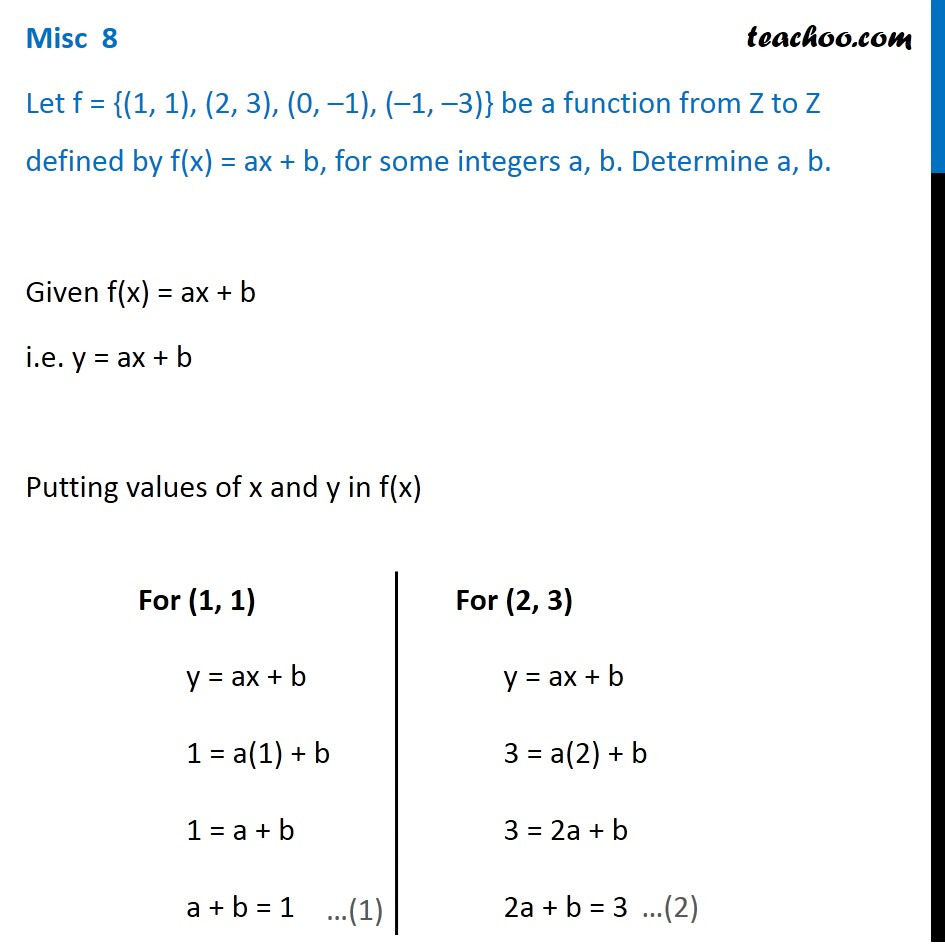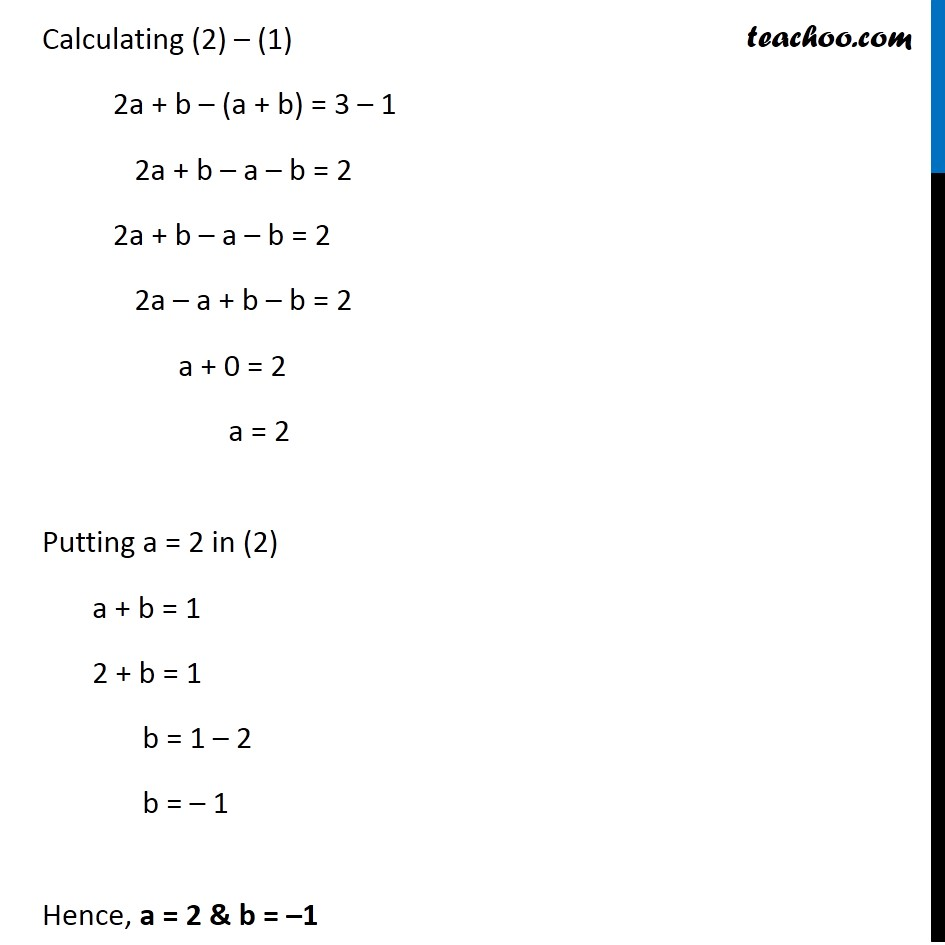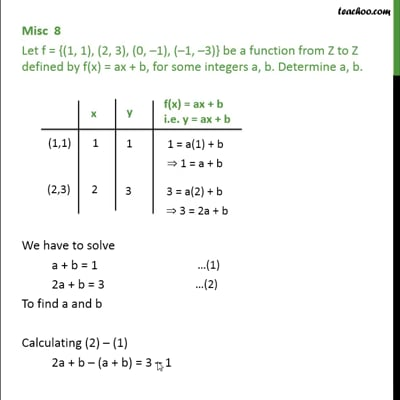Miscellaneous

Chapter 2 Class 11 Relations and Functions
Serial order wiseThis video is only available for Teachoo black users

Solve all your doubts with Teachoo Black (new monthly pack available now!)

### Transcript

Misc 8 Let f = {(1, 1), (2, 3), (0, –1), (–1, –3)} be a function from Z to Z defined by f(x) = ax + b, for some integers a, b. Determine a, b. Given f(x) = ax + b i.e. y = ax + b Putting values of x and y in f(x) For (1, 1) y = ax + b 1 = a(1) + b 1 = a + b a + b = 1 For (2, 3) y = ax + b 3 = a(2) + b 3 = 2a + b 2a + b = 3 Calculating (2) – (1) 2a + b – (a + b) = 3 – 1 2a + b – a – b = 2 2a + b – a – b = 2 2a – a + b – b = 2 a + 0 = 2 a = 2 Putting a = 2 in (2) a + b = 1 2 + b = 1 b = 1 – 2 b = – 1 Hence, a = 2 & b = –1